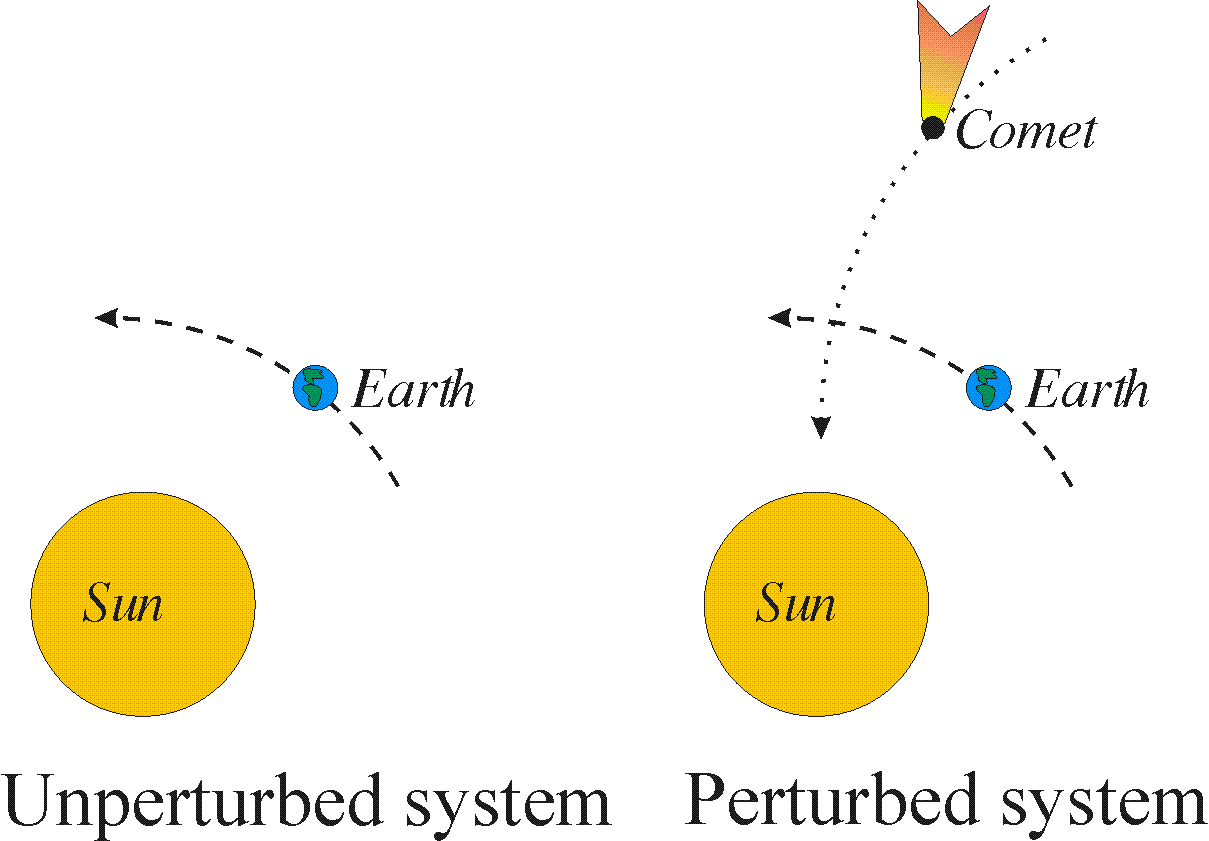# 1. Introduction..Let the mass of the earth be m. Consider the motion of the earth around the sun in an ideal case, where we have no influences from other celestial bodies. The motion y=y(t) is governed by Newton’s lawWe now imagine the motion being perturbed by a comet passing close to the earth. Then the perturbed system will have an extra termIt is not unreasonable that the solution of the perturbed problem might be on the formwhere y0(t) is the solution of the original problem and the rest are correction terms.

Remark: The notationis often used for first, second,… order derivatives of a function y=y(t)depending on the time t.